# Correlating Data

Problem

You're working with a multivariable problem and want to compute the correlation coefficient between variables.

Solution

Use Excel's built-in functions CORREL or PEARSON, or use the Correlation tool available in the Analysis ToolPak.

Discussion

In Recipe 8.4, I show you how to perform linear regression where the dependent variable depends on more than one independent variable. The example I discuss in that recipe involves six independent variables. This is a typical example where it's prudent to assess the correlation between independent variables before conducting the regression analysis. This way you can avoid using highly correlated (or colinear) independent variables, which can give you trouble.

Excel provides two built-in functions that allow you to compute the Pearson product-moment correlation coefficients between variables. These functions are CORREL and PEARSON and, according to Excel's help documents, they perform exactly the same calculation. (I've no idea why there are two functions to do the same thing.)

Figure 5-8 shows the data from the multiple linear regression example I discuss in Chapter 8. For now, I'll show you how to examine the correlation between independent variablesthe x variablesusing Excel's correlation functions as well as the Analysis ToolPak.

To compute the correlation coefficient between variables x1 and x2, you can use the cell formula =CORREL(E7:E22,F7:F22) or =PEARSON(E7:E22,F7:F22). They both produce the same results, which is 0.991589. Clearly, these two variables are positively correlated and in a regression analysis, for example, you would probably exclude one of these variables from the curve fit. You can compute correlation coefficients between the other variables in a similar manner.

Figure 5-8. Multiple linear regression data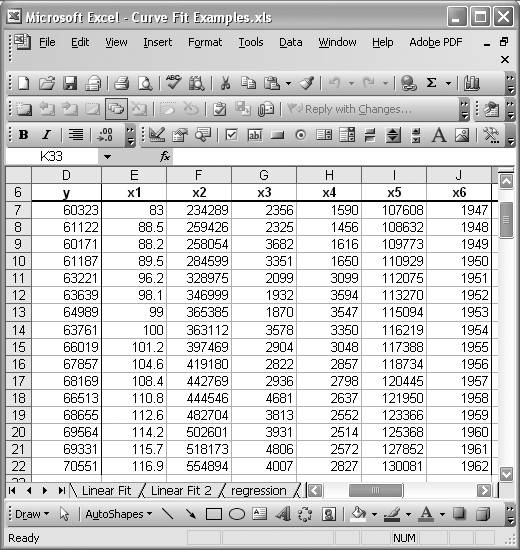To save some time when dealing with numerous variables, you might consider using the Correlation tool available in the Analysis ToolPak. Select Tools [Image_Link]/images/U2192.jpg border=0> Data Analysis from the main menu bar to open the Data Analysis dialog box shown in Figure 5-9.

Figure 5-9. Data Analysis Correlation tool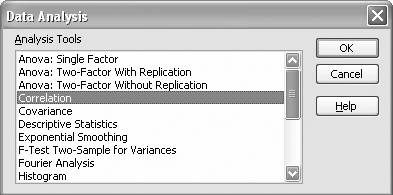Select the Correlation tool and press OK to open the Correlation tool dialog box shown in Figure 5-10.

Figure 5-10. Correlation tool dialog boxThe Correlation tool allows you to select multiple datasets at once; it will compute the correlation coefficients between all the datasets. For example, in Figure 5-10 I entered cells E6 through J22 in the Input Range. This cell range includes all of the x variables shown in Figure 5-8. I included the header labels in the input range, so I checked the "Labels in first row" option. Further, I specified cell M7 (on the same worksheet) as the starting cell where I'd like the output displayed. Figure 5-11 shows the results.

Figure 5-11. Correlation results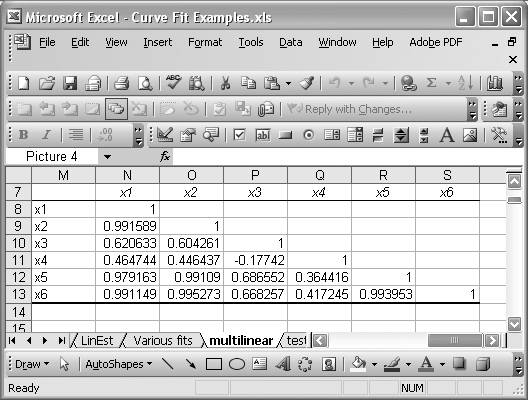This table presents the correlation coefficient between each given variable and every other variable. It also correlates each variable with itself, which of course results in a correlation coefficient of 1 (as you can see in Figure 5-11).

Sometimes it's useful to compute coefficients of determination instead of correlation coefficients alone. To compute a coefficient of determination, simply square the correlation coefficient. For example, if cell N9 contains the correlation coefficient between variables x1 and x2, then the formula =N9^2 would yield the coefficient of determination.

There are other types of correlation coefficients common in statistical analysis. For example, Phi, rank biserial, point biserial, and Spearman rank coefficients are common, in addition to the Pearson coefficient. Excel does not have built-in support for these other correlation coefficients; however, you can certainly set up a spreadsheet to calculate these coefficients.

For example, you can readily prepare a spreadsheet to compute the Spearman rank correlation coefficient between variables x1 and x2 from the previous example. The Spearman coefficient requires you to convert the raw data to ranks first. You can achieve this conversion using Excel's built-in RANK function (see Recipe 5.5). Figure 5-12 shows a simple spreadsheet I set up to compute the Spearman rank correlation coefficient between the variables x1 and x2 from the previous example.

The first two columns in the table contain the raw data. The third and fourth columns contain the rank of each data point. I used the RANK function for these calculations. For example, cell E41 contains the cell formula =RANK(C41,\$C\$41:\$C\$56,1), which computes the rank for the first x1 value, assuming the data are sorted in ascending order (see Recipe 5.5).

The formula for computing the Pearson rank correlation coefficient is: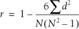Here, d is the difference in ranks between corresponding values for both x1 and x2, and N is the number of data points. You can compute N using the COUNT function. Cell D58 contains the formula =COUNT(D41:D56) which returns a value of 16 for the number of points.

To compute d2, I set up another column containing formulas like =(E41-F41)^2. The results are shown in the last column of the table shown in Figure 5-12.

Cell G57 computes the sum of d2 values using the formula =SUM(G41:G56). Cell G59 computes the N(N2 - 1) term using the formula =(D58*(D58^2-1)). And finally, the correlation coefficient is computed in cell G60 using the formula =1-6*G57/G59. The resulting correlation coefficient is 0.997.

Figure 5-12. Spearman rank correlation coefficient calculation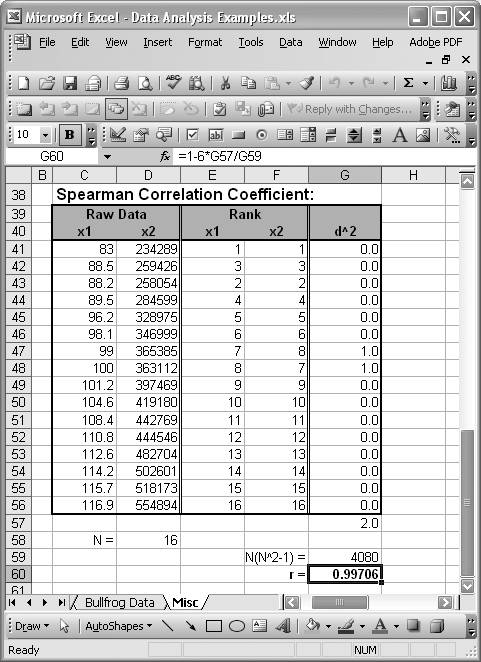Take a look at Recipe 8.4 to see the resulting regression model for this example dataset. Chapter 8 also discusses coefficients of determination in the context of least-squares curve fitting.Excel Scientific and Engineering Cookbook (Cookbooks (OReilly))
ISBN: 0596008791
EAN: 2147483647
Year: N/A
Pages: 206
Authors: David M Bourg